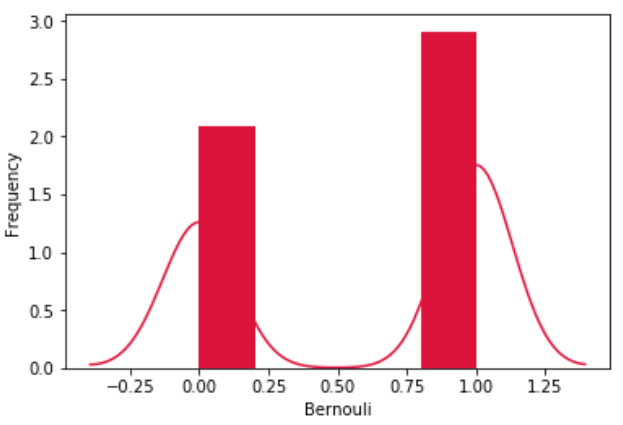# Python - Bernoulli Distribution

The Bernoulli distribution is a special case of the Binomial distribution where a single experiment is conducted so that the number of observation is 1. So, the Bernoulli distribution therefore describes events having exactly two outcomes.

We use various functions in numpy library to mathematically calculate the values for a bernoulli distribution. Histograms are created over which we plot the probability distribution curve.

```from scipy.stats import bernoulli
import seaborn as sb

data_bern = bernoulli.rvs(size=1000,p=0.6)
ax = sb.distplot(data_bern,
kde=True,
color='crimson',
hist_kws={"linewidth": 25,'alpha':1})
ax.set(xlabel='Bernouli', ylabel='Frequency')
```

Its output is as follows −## Useful Video Courses

Video

#### Python Online Training

187 Lectures 17.5 hours

Video

#### Python Essentials Online Training

55 Lectures 8 hours

Video

#### Learn Python Programming in 100 Easy Steps

136 Lectures 11 hours

Video

#### Python with Data Science

Best Seller

75 Lectures 13 hours

Video

#### Python 3 from scratch to become a developer in demand

Best Seller

70 Lectures 8.5 hours

Video

#### Python Data Science basics with Numpy, Pandas and Matplotlib

Most Popular

63 Lectures 6 hours